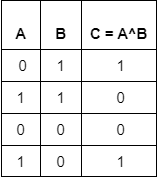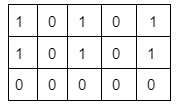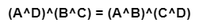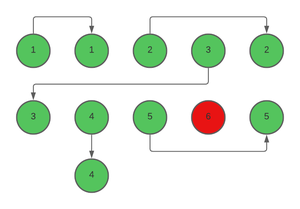GFG App
Open AppBrowser
Continue

In a party of N people, each person is denoted by an integer. Couples are represented by the same number. Find out the only single person at the party of couples.

Examples:

Input: N = 5, arr = {1, 2, 3, 2, 1}
Output: 3
Explanation: Only the number 3 is single.

Input: N = 11, arr = {1, 2, 3, 5, 3, 2, 1, 4, 5, 6, 6}
Output: 4
Explanation: 4 is the only single.

Naive approach: To solve the problem follow the below steps:

• Sort the array of integers.
• Now, traverse the array by screening two elements at once. We do this because numbers present in a couple would be present in a group of two and would appear consecutively in a sorted array.
• When we come across a group of two elements that are not the same, the minimum of the two elements or the first element of the group in the sorted array is the single element in the party of couples.

Representation of the above approach:

For e.g., we take an array of integers = {1, 1, 2, 3, 2, 3, 4, 5, 6, 5, 4}

After sorting the array becomes = {1, 1,  2, 2,  3, 3,  4, 4,   5, 5,  6}

As we can see, 6 is the single element that doesn’t have its pair.

The time complexity of this algorithm will be O(N log N), as we are sorting the array of numbers first which is the most expensive operation.

## Alone in Couple using Bitwise Operator:

To understand the solution to this problem, first, we have to understand the Bitwise Operator, XOR. The truth table of XOR is given below for reference:Truth Table for XOR

To quickly recap a really useful property of XOR: When operated on two same numbers, it returns 0. This property can be easily deduced by the truth table given above. When a number will be XOR’ed with itself the binary representation of both A and B in the above truth table would be the same and result in 0 in bits of all positions.

For Eg:A number XOR’ed with itself always returns 0.

At the party, we have two numbers that are identical to each other, which belong to the couples. If they are XOR’ed with each other, they will return the answer as 0.   To have a full understanding of the solution, we need to know that XOR is associative, i.e., the order of grouping doesn’t matter when multiple XOR operations are done. For e.g.The order of grouping doesn’t change the result of the expression.

So, if we XOR all the numbers of attendees with each other, we will obtain the number which doesn’t have its pair.
A pictorial representation of the same concept is given with the example below:The duplicate numbers when XOR’ed will cancel each other irrespective of the order because of the associative property of XOR.

Follow the steps to solve the problem:

• Declare an integer variable ‘x‘ with the starting value as 0.
• Iterate through the array of numbers representing the attendees, and
• Keep updating the ‘x’ with each iteration after performing XOR with the current element.
• The integer ‘x‘ represents the single Attendee at the party.

Below is the implementation for the above approach:

## C++

```// C++ code for the above approach:
#include <bits/stdc++.h>
using namespace std;

void solve(int* arr, int n)
{
// Initialize a variable with 0 value,
// as A^0 = A.
int x = 0;
for (int i = 0; i < n; i++) {
x = x ^ arr[i];
}

// The number remaining at the end
// will be the single number which
// doesn't have it's pair to make it 0
cout << x;
}

// Drivers code
int main()
{

// Declaring the attendees of the party
// with only one single candidate
int arr[] = { 1, 2, 3, 5, 3, 2, 1, 4, 5, 6, 6 };

// The number 6 is the only
// single attendee.
int n = sizeof(arr) / sizeof(int);

// Function Call
solve(arr, n);

return 0;
}```

## Java

```// JAVA function to implement above approach
import java.util.*;
class GFG {

static void solve(int[] arr, int n)
{
// Initialize a variable with 0 value,
// as A^0 = A.
int x = 0;
for (int i = 0; i < n; i++) {
x = x ^ arr[i];
}

// The number remaining at the end
// will be the single number which
// doesn't have it's pair to make it 0
System.out.print(x);
}

// Driver Function
public static void main(String[] args)
{

// Declaring the attendees of the party
// with only one single candidate
int arr[] = { 1, 2, 3, 5, 3, 2, 1, 4, 5, 6, 6 };

// The number 6 is the only
// single attendee.
int n = arr.length;

// Function Call
solve(arr, n);
}
}

// This code is contributed by sanjoy_62.```

## Python3

```# Python function to implement the above approach
def solve(arr, n):

# Initialize a variable with 0 value as A^0 = A.
x = 0
for i in range(n):
x = x ^ arr[i]

# The number remaining at the end will be the single
# number which doesn't have it's pair to make it 0
print(x)

# Declaring the attendees of the party with only one
# single candidate
arr = [1, 2, 3, 5, 3, 2, 1, 4, 5, 6, 6]

# The number 6 is the only single attendee.
n = len(arr)

# Function call
solve(arr, n)

# This code is contributed by lokeshmvs21.```

## C#

```// C# code to implement the above approach
using System;
public class GFG
{

static void solve(int[] arr, int n)
{

// Initialize a variable with 0 value,
// as A^0 = A.
int x = 0;
for (int i = 0; i < n; i++) {
x = x ^ arr[i];
}

// The number remaining at the end
// will be the single number which
// doesn't have it's pair to make it 0
Console.WriteLine(x);
}

// Driver Code
public static void Main(string[] args)
{

// Declaring the attendees of the party
// with only one single candidate
int[] arr = { 1, 2, 3, 5, 3, 2, 1, 4, 5, 6, 6 };

// The number 6 is the only
// single attendee.
int n = arr.Length;

// Function Call
solve(arr, n);
}
}

// This code is contributed by code_hunt.```

## Javascript

```// javascript code for the above approach:

function solve( arr,  n)
{
// Initialize a variable with 0 value,
// as A^0 = A.
let x = 0;
for (let i = 0; i < n; i++) {
x = x ^ arr[i];
}

// The number remaining at the end
// will be the single number which
// doesn't have it's pair to make it 0
console.log(x);
}

// Drivers code

// Declaring the attendees of the party
// with only one single candidate
let arr = [ 1, 2, 3, 5, 3, 2, 1, 4, 5, 6, 6 ];

// The number 6 is the only
// single attendee.
let n = arr.length;

// Function Call
solve(arr, n);

// this code is contributed by ksam24000```

## PHP

```<?php
function solve(\$arr, \$n)
{
// Initialize a variable with 0 value,
// as A^0 = A.
\$x = 0;
for (\$i = 0; \$i < \$n; \$i++) {
\$x = \$x ^ \$arr[\$i];
}

// The number remaining at the end
// will be the single number which
// doesn't have it's pair to make it 0
echo \$x;
}

// Declaring the attendees of the party
// with only one single candidate
\$arr = array(1, 2, 3, 5, 3, 2, 1, 4, 5, 6, 6);

// The number 6 is the only
// single attendee.
\$n = count(\$arr);

// Function Call
solve(\$arr, \$n);
//This code is contributed by Kanishka Gupta
?>```
Output

`4`

Time complexity: O(N), where N is the size of the array as we are traversing through the array once.
Auxiliary Space: O(1)

My Personal Notes arrow_drop_up
Related Tutorials